Homework Help Question & Answers

# Write the rate law for the reaction. Hydrolysis of PNA OH high pH +H2O HO NO2...

Write the rate law for the reaction.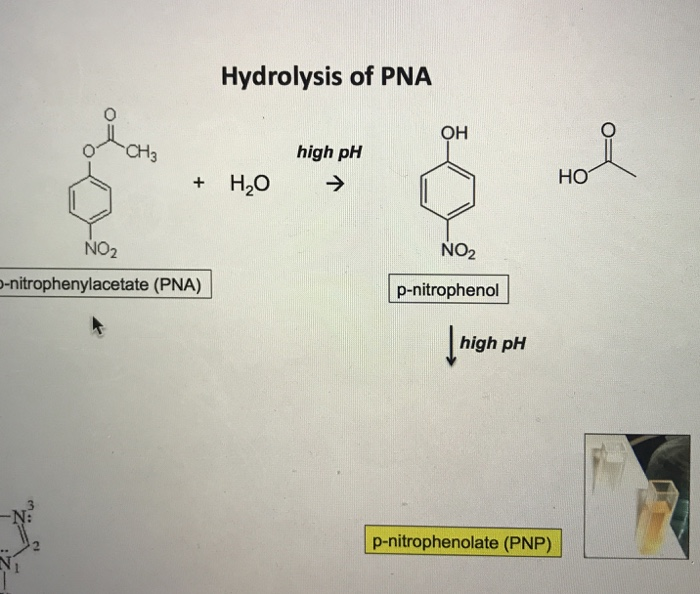Hydrolysis of PNA OH high pH +H2O HO NO2 NO2 -nitrophenylacetate (PNA) p-nitrophenol high pH p-nitrophenolate (PNP)

#### Homework Answers

Answer #1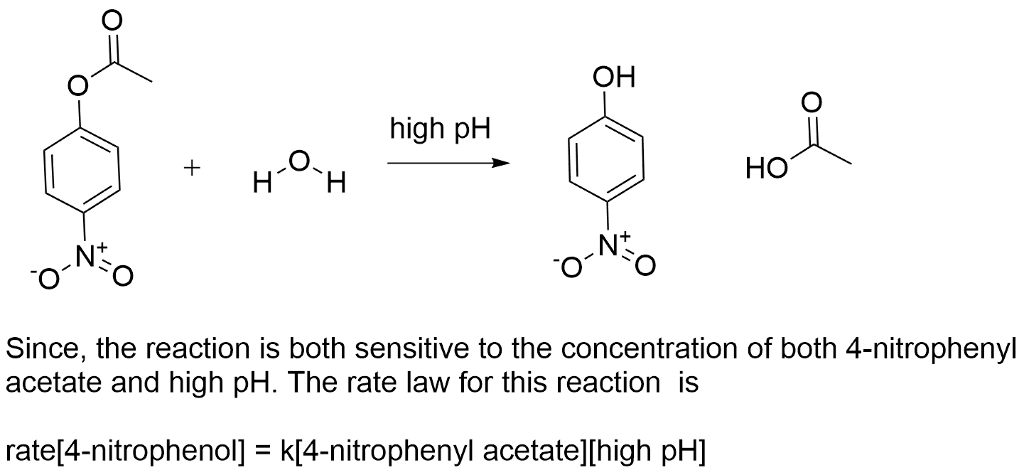Know the answer?
Your Answer:

#### Post as a guest

Your Name:

What's your source?

#### Earn Coin

Coins can be redeemed for fabulous gifts.

Not the answer you're looking for? Ask your own homework help question. Our experts will answer your question WITHIN MINUTES for Free.
Similar Homework Help Questions
• ### 3. Cr** is acidic by virtue of the following hydrolysis reaction: Cr3+ + H2O + Cr(OH)2+...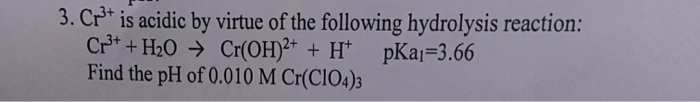3. Cr** is acidic by virtue of the following hydrolysis reaction: Cr3+ + H2O + Cr(OH)2+ + H+ pka;=3.66 Find the pH of 0.010 M Cr(ClO4)3

• ### A possible mechanism for the overall reaction, ОН + NO HNO in the presence of N, is: (HO... NO,) (HO...NO2) OH NO N...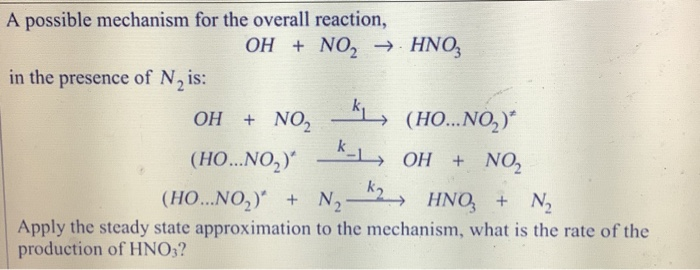A possible mechanism for the overall reaction, ОН + NO HNO in the presence of N, is: (HO... NO,) (HO...NO2) OH NO NO2 ОН (HO...NO,) N2 HNO N Apply the steady state approximation to the mechanism, what is the rate of the production of HNO,?

• ### 8. Find the rate law of the reaction: keff 3HNO2 H2O + 2NO + H+ +...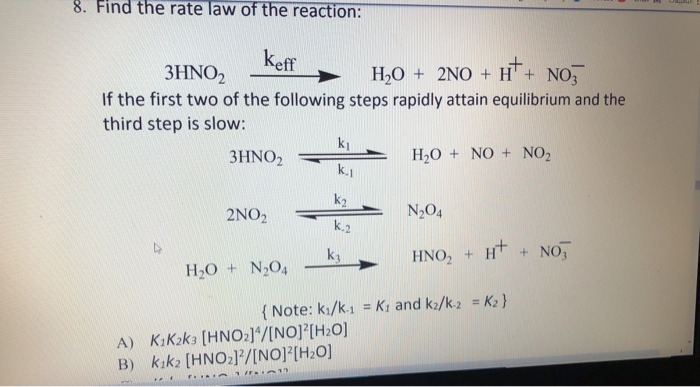8. Find the rate law of the reaction: keff 3HNO2 H2O + 2NO + H+ + NO, If the first two of the following steps rapidly attain equilibrium and the third step is slow: 3HNO2 H2O + NO + NO2 2N02 N204 HNO3 + H+ + NO; HO + N204 { Note: ki/k-1 = Ky and k2/k2 = K2} A) K.Kak3 [HNO2]/[NO] [H20] B) Kik2 [HNO2]/[NO] [H2O] 1 + FRA 1

• ### Q2. Complete the following base hydrolysis reaction of ester. (1) NaOH (2) (H2O) Q3. Write down...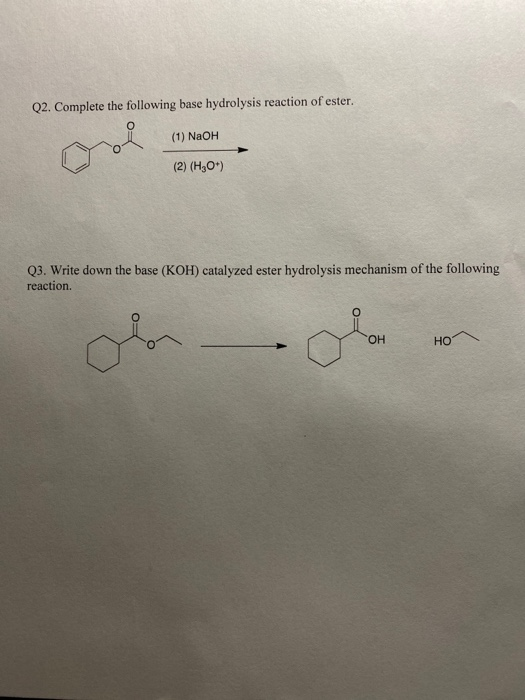Q2. Complete the following base hydrolysis reaction of ester. (1) NaOH (2) (H2O) Q3. Write down the base (KOH) catalyzed ester hydrolysis mechanism of the following reaction. OH ol 우

• ### Fenton Reaction HO3 + Felt ki Fe+ + + + HO. Fe+ + H2O, k2Fe+ + OH- + OH HO, + Fel+ + Fe2+ + H OH + Fe+ K OH + Fe+ O...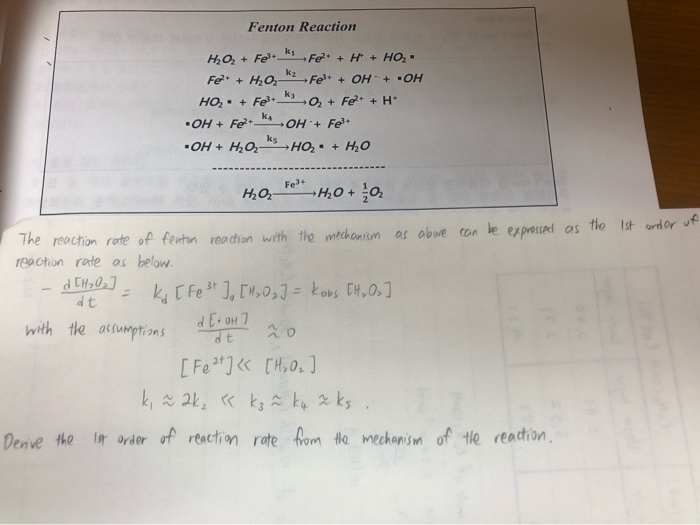Fenton Reaction HO3 + Felt ki Fe+ + + + HO. Fe+ + H2O, k2Fe+ + OH- + OH HO, + Fel+ + Fe2+ + H OH + Fe+ K OH + Fe+ OH + H2O, SHO, . + H2O HQ Felt H₂O + 6 as the 1st ordor of The reaction rate of fenton reaction with the mechanism as above can be expressed reaction rate as below. - d CH₂02] - K [Fe 3+ ] [H₂O₃] = kobs CH,...

• ### Choose the products of the reaction shown below. Pay attention to details! HOCH OH HO hydrolysis...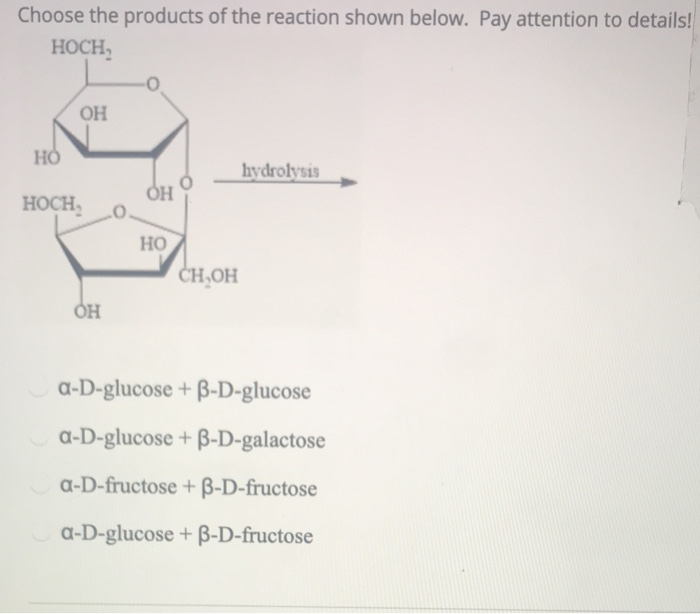Choose the products of the reaction shown below. Pay attention to details! HOCH OH HO hydrolysis OH HOCH HO CHOH OH a-D-glucose + B-D-glucose a-D-glucose + B-D-galactose a-D-fructose + B-D-fructose a-D-glucose + B-D-fructose

• ### The hydrolysis of tert-butyl chloride occurs according to the following equation and rate law: C4H9Cl +...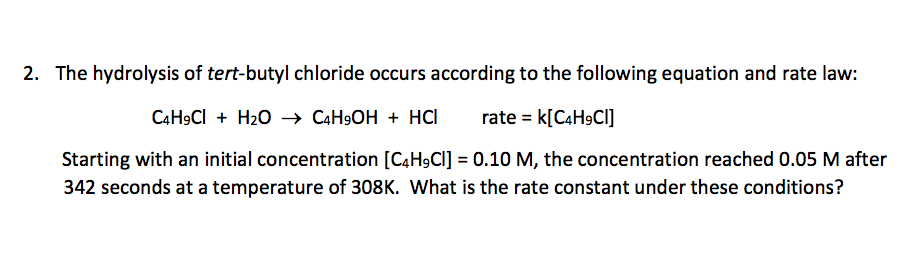The hydrolysis of tert-butyl chloride occurs according to the following equation and rate law: C4H9Cl + H2O  C4H9OH + HCl rate = k[C4H9Cl] Starting with an initial concentration [C4H9Cl] = 0.10 M, the concentration reached 0.05 M after 342 seconds at a temperature of 308K. What is the rate constant under these conditions? 2. The hydrolysis of tert-butyl chloride occurs according to the following equation and rate law: C&HgCl + H2O + C4H,OH + HCI rate = k[C4H9CI] Starting...

• ### 8. For the reaction HCN (%) + H2O (1) HO*)+CN) how would adding OH-shift the equilibrium?...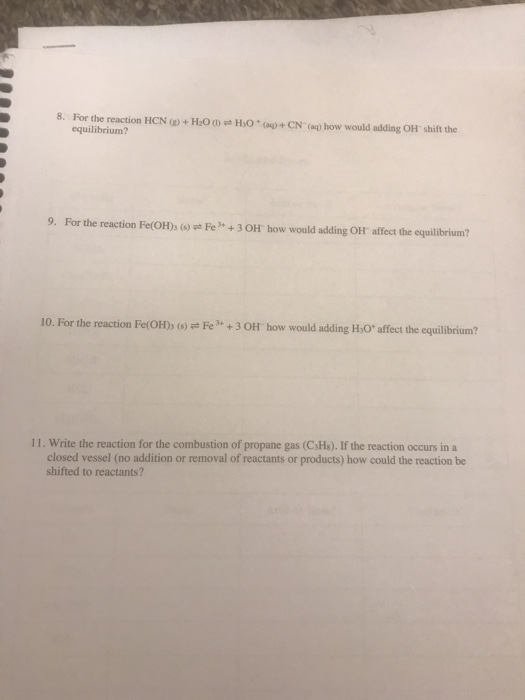8. For the reaction HCN (%) + H2O (1) HO*)+CN) how would adding OH-shift the equilibrium? 9. For the reaction Fe(OH)3 (8) Fe' + 3 OH how would adding OH" affect the equilibrium? 10. For the reaction Fe(OH)3 () + Fe 3+ + 3 OH how would adding H30affect the equilibrium? 11. Write the reaction for the combustion of propane gas (CsHx). If the reaction occurs in a closed vessel (no addition or removal of reactants or products) how could...

• ### Isolation Method of Rate Law Determination Consider the base hydrolysis of an ester RCOOR' + OH-→...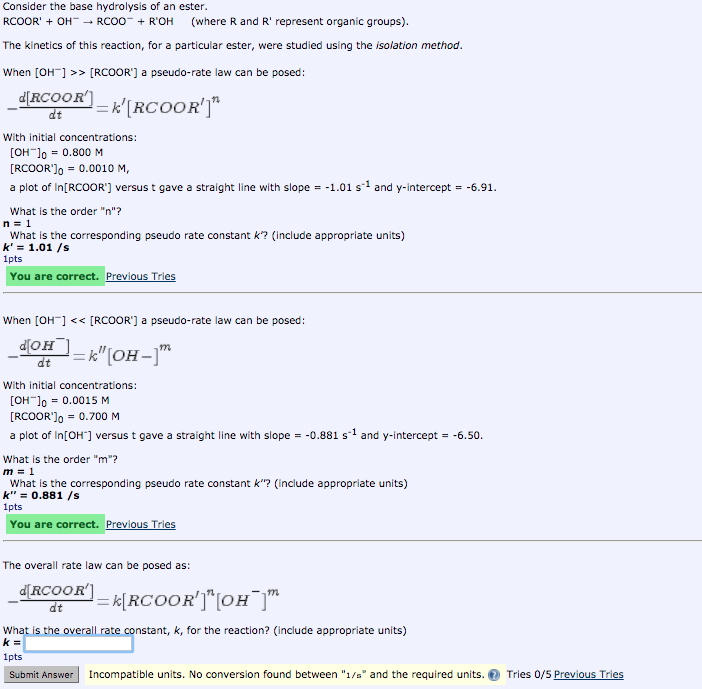Isolation Method of Rate Law Determination Consider the base hydrolysis of an ester RCOOR' + OH-→ RCOO-+ R'OH (where R and R"represent organic groups) The kinetics of this reaction, for a particular ester, were studied using the isolation method When [OH>> [RCOOR'] a pseudo-rate law can be posed: With initial concentrations: [OH-]0 = 0.800 M [RCOOR']0 = 0.0010 M, a plot of In[RCOORT versus t gave a straight line with slope =-1.01 s-1 and y-intercept =-6.91 What is the order...

• ### 5. Provide the product of the following reaction H A. H- -OH Br2, H2O НО- -H...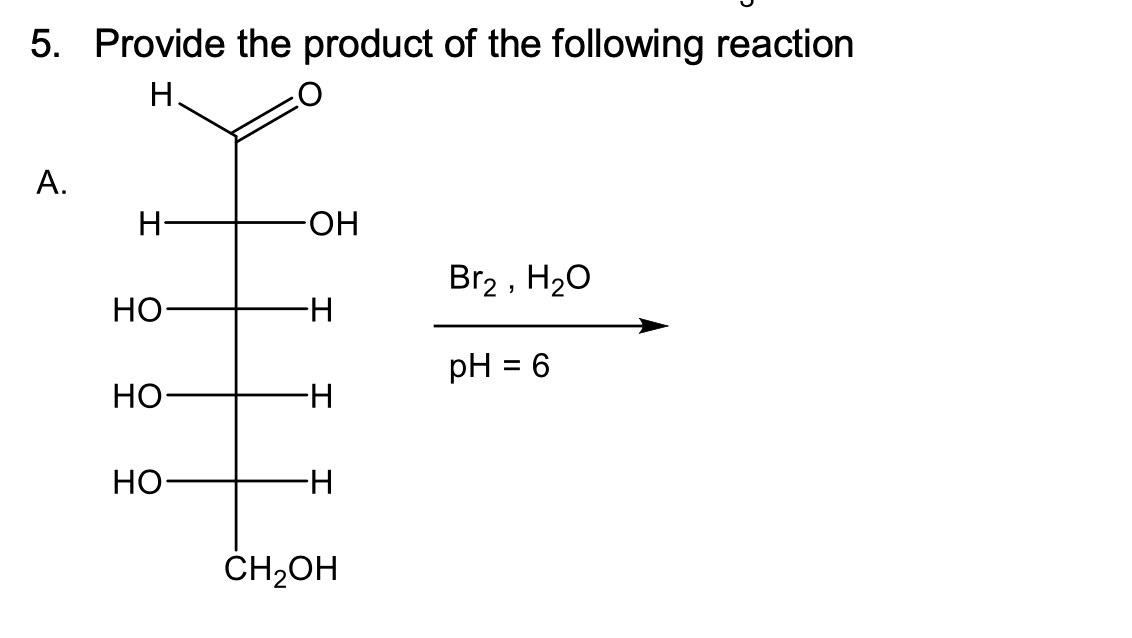5. Provide the product of the following reaction H A. H- -OH Br2, H2O НО- -H pH = 6 HO -H HO -H CH2OH